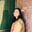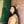Trusted answers to developer questions
Trusted Answers to Developer Questions

Related Tags

python
numpy

# What is numpy.subtract() in Python?Umme Ammara

Grokking Modern System Design Interview for Engineers & Managers

Ace your System Design Interview and take your career to the next level. Learn to handle the design of applications like Netflix, Quora, Facebook, Uber, and many more in a 45-min interview. Learn the RESHADED framework for architecting web-scale applications by determining requirements, constraints, and assumptions before diving into a step-by-step design process.

Python’s numpy.subtract() method subtracts two arrays element-wise.

## Syntax

numpy.subtract() is declared as shown below:

numpy.subtract(x1, x2, /, out=None, *, where=True, casting='same_kind', order='K', dtype=None, subok=True[, signature, extobj]) = <ufunc 'subtract'>


A universal function (ufunc) is a function that operates on ndarrays in an element-by-element fashion. The subtract() method is a universal function.

## Parameters

The numpy.subtract() method takes the following compulsory parameters:

• x1 and x2 [array-like] - arrays that need to be subtracted. If the shapethe shape of an array is the number of elements in each dimension of x1 and x2 is different, they must be broadcastable to a common shape for representing the output.

The numpy.subtract() method takes the following optional parameters:

 Parameter Description out Represents the location into which the output of the method is stored. If not provided or None, a freshly-allocated array is returned. where True value indicates that a universal function should be calculated at this position. casting Controls the type of datacasting that should occur. The same_kind option indicates that safe casting or casting within the same kind should take place. order Controls the memory layout order of the output function. The option K means reading the elements in the order they occur in memory. dtype Represents the desired data type of the array. subok Decides if subclasses should be made or not. If True, subclasses will be passed through.

## Return value

numpy.subtract() returns the difference of the two arrays element-wise. The return type is either ndarray or scalar depending on the input type.

## Examples

The examples below show the different ways numpy.subtract() is used in Python.

### Subtraction of two numbers

The code below outputs the difference of two numbers, 17.5 and 12. The result is shown below:

import numpy as npa = 17.5b = 12result = np.subtract(a,b)print (result)

### Subtraction of two arrays

The example below shows the result of subtracting two arrays arr1 and arr2:

import numpy as nparr1 = np.array([20,30,40])arr2 = np.array([2,3,4])result = np.subtract(arr1,arr2)print (result)

The example below shows the result of subtracting two arrays arr3 and arr4:

import numpy as nparr3 = np.array([[20,30,40], [-2,-3,-4]])arr4 = np.array([[-2,-3,-4], [30,40,50]])result = np.subtract(arr3,arr4)print (result)

RELATED TAGS

python
numpy

CONTRIBUTORUmme Ammara